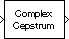Documentation

### This is machine translation

Mouseover text to see original. Click the button below to return to the English version of the page.

# Complex Cepstrum

Compute complex cepstrum of input

## Library

Transforms

`dspxfrm3`## Description

The Complex Cepstrum block computes the complex cepstrum of each column in the real-valued M-by-N input matrix, u. The block treats each column of the input as an independent channel containing M consecutive samples. The block always processes unoriented vector inputs as a single channel, and returns the result as a length-M column vector. The block does not accept complex-valued inputs.

The input is altered by the application of a linear phase term so that there is no phase discontinuity at ±π radians. That is, each input channel is independently zero padded and circularly shifted to have zero phase at π radians.

The output is a real Mo-by-N matrix, where Mo is specified by the FFT length parameter. Each output column contains the length-Mo complex cepstrum of the corresponding input column.

```y = cceps(u,Mo) % Equivalent MATLAB code ```

When you select the Inherit FFT length from input port dimensions check box, the output frame size matches the input frame size (Mo = M).

The output port rate is the same as the input port rate.

## Parameters

Inherit FFT length from input port dimensions

When you select this check box, the output frame size matches the input frame size.

FFT length

The number of frequency points at which to compute the FFT, which is also the output frame size, Mo. This parameter is visible only when you clear the Inherit FFT length from input port dimensions check box.

## Supported Data Types

• Double-precision floating point

• Single-precision floating point

 DCT DSP System Toolbox FFT DSP System Toolbox Real Cepstrum DSP System Toolbox `cceps` Signal Processing Toolbox